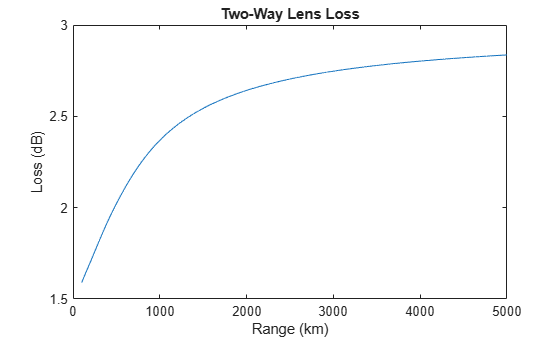# lenspl

Calculate loss due to tropospheric lens effect

## Syntax

``L = lenspl(R,H,EL)``
``L = lenspl(___,Name,Value)``

## Description

example

````L = lenspl(R,H,EL)` calculates the one-way loss due to the tropospheric lens effect using the International Telecommunication Union (ITU) standard atmospheric model known as the mean annual global reference atmosphere (MAGRA), which approximates the U.S. Standard Atmosphere 1976 with insignificant relative error. The variation in refraction versus altitude makes the atmosphere act like a lens with loss independent of frequency. Rays leaving an antenna are refracted in the troposphere and the energy radiated within some angular extent is distributed over a slightly greater angular sector, thereby reducing the energy density relative to propagation in a vacuum.```
````L = lenspl(___,Name,Value)` specifies options using one or more name-value arguments in addition to the input arguments in the previous syntax.```

## Examples

collapse all

Calculate the two-way lens loss curve for a radar platform at sea level at an elevation angle of `0.03` deg over a slant range of `0.1` to `5.0` km.

```h = 0; % m el = 0.03; % deg R = (100:5000).*1e3; % m L = 2*lenspl(R,h,el); % Factor of 2 for two-way propagation ```

Plot the lens loss against the slant range.

```plot(R.*1e-3,L); xlabel('Range (km)'); ylabel('Loss (dB)'); title('Two-Way Lens Loss');```## Input Arguments

collapse all

Slant range, specified as a scalar or an N-length vector. Units are in meters.

Example: `0.5`

Data Types: `single` | `double`

Mean sea level (MSL) altitude of the radar platform, specified as a scalar from `0` to `100` km. Values outside the specified range result in `NaN` output. Units are in meters.

Example: `200e3`

Data Types: `single` | `double`

Elevation angle of the propagation path, specified as a positive scalar. Units are in degrees.

Example: `10`

Data Types: `single` | `double`

### Name-Value Pair Arguments

Specify optional comma-separated pairs of `Name,Value` arguments. `Name` is the argument name and `Value` is the corresponding value. `Name` must appear inside quotes. You can specify several name and value pair arguments in any order as `Name1,Value1,...,NameN,ValueN`.

Example:

Standard ground-level water vapor density, specified as a positive scalar. Applicable only for the default standard model (MAGRA). Units are in grams per meter cubed.

Data Types: `single` | `double`

Altitude above mean sea level (MSL), specified as a scalar. Applicable only for the default standard model (MAGRA). For dry atmosphere conditions, set to `6e3` m. Units are in meters.

Data Types: `single` | `double`

Reference latitude model, specified as one of these.

ModelDescription
`'Standard'`(default)

This model is the mean annual global reference atmosphere (MAGRA) that reflects the mean annual temperature and pressure averaged across the world.

`'Low'`

This model is for low latitudes less than `22` degrees, where there is little seasonal variation.

`'Mid'`

This model is for mid latitudes between `22` and `45` degrees with seasonal profiles for `'Summer'` and `'Winter'`, which can be specified using the `'Season'` name-value argument.

`'High'`

This model is for high latitudes greater than `45` degrees with seasonal profiles for `'Summer'` and `'Winter'`, which can be specified using the `'Season'` name-value argument.

Season for the `'Mid'` and `'High'` latitude models, specified as `'Summer'` or `'Winter'`. Other models ignore this input. Defaults to `'Summer'`.

Custom atmospheric measurements for the calculation of the refractive index, specified as an N-by-`4` matrix, where N corresponds to the number of altitude measurements. The first column is the atmospheric temperature in kelvins, the second column is the atmospheric pressure in hPa, the third column is the water vapor density in g/m3, and the fourth column is the MSL altitude of the measurements in meters. When you use a custom model, all other name-value arguments are ignored and the output refractive index is applicable for the input height.

Note

The model used by `lenspl` assumes geometrical optics conditions, as a result anomalous propagation like ducting and subrefraction cannot be present in provided measurements. If atmospheric measurements evidencing ducting and subrefraction are provided, this function throws an error.

Data Types: `single` | `double`

## Output Arguments

collapse all

The one-way lens loss, returned as a scalar or M-length vector. Units are in decibels.

## Extended Capabilities

### External Websites

Introduced in R2021a